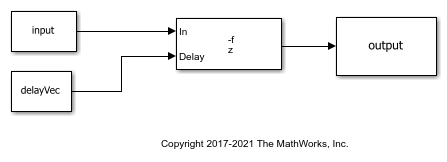Main Content

# Delay Signal Using Multitap Fractional Delay

Delay the input signal using the Variable Fractional Delay block. Each delay value is unique and can vary from sample to sample within a frame, and can vary across channels. You can compute multiple delayed versions of the same input signal concurrently by passing a delay input with the appropriate dimension.

Consider the input to be a random signal with one channel and a frame size of 10. Apply a delay of 4.8 and 8.2 samples concurrently.

Open the model.

```model = 'MultitapFractionalDelay'; open_system(model) ```Run the model.

```input = randn(10,1) %#ok ```
```input = 0.5377 1.8339 -2.2588 0.8622 0.3188 -1.3077 -0.4336 0.3426 3.5784 2.7694 ```
```delayVec = [4.8 8.2]; %#ok sim(model) display(output) ```
```output = 0 0 0 0 0 0 0 0 0.1075 0 0.7969 0 1.0153 0 -1.6346 0 0.7535 0.4301 -0.0065 1.5746 ```

Each channel in the output is delayed by 4.8 and 8.2 samples, respectively. The block uses the 'Linear' interpolation method to compute the delayed value. For more details, see 'Algorithms' in the Variable Fractional Delay block page.

For the same delay vector, if the input has 2 channels, each element of the delay vector is applied on the corresponding channel in the input.

```input = randn(10,2); ```
```sim(model); display(output); ```
```output = 0 0 0 0 0 0 0 0 -0.2700 0 -0.4729 0 2.5730 0 0.5677 0 0.0925 0.5372 0.5308 -0.8317 ```

To compute multiple delayed versions of the two-dimensional input signal, pass the delay vector as a three-dimensional array. The third dimension contains the taps or delays to apply on the signal. If you pass a non-singleton third dimension (1-by-1-by-P), where P represents the number of taps, the same tap is applied across all the channels. Pass the delays [4.8 8.2] in the third dimension.

```clear delayVec; delayVec(1,1,1) = 4.8; delayVec(1,1,2) = 8.2; %#ok whos delayVec ```
``` Name Size Bytes Class Attributes delayVec 1x1x2 16 double ```

`delayVec` is a 1-by-1-by-2 array. Pass the two-dimensional input to the `Variable Fractional Delay` block with this delay vector.

```sim(model) display(output) ```
```output(:,:,1) = 0 0 0 0 0 0 0 0 -0.2700 0.1343 -0.4729 0.2957 2.5730 -0.8225 0.5677 0.8998 0.0925 1.4020 0.5308 0.5981 output(:,:,2) = 0 0 0 0 0 0 0 0 0 0 0 0 0 0 0 0 -1.0799 0.5372 2.1580 -0.8317 ```
```whos output ```
``` Name Size Bytes Class Attributes output 10x2x2 320 double ```

`output(:,:,1)` represents the input signal delayed by 4.8 samples. `output(:,:,2)` represents the input signal delayed by 8.2 samples. The same delay is applied across all the channels.

In addition, if you pass a non-singleton second dimension (1-by-L-by-P), where L is the number of input channels, taps vary across channels. Apply the delay vectors [2.3 3.5] and [4.4 5.6] to compute the two delayed versions of the input signal.

```clear delayVec; delayVec(1,1,1) = 2.3; delayVec(1,2,1) = 3.5; delayVec(1,1,2) = 4.4; delayVec(1,2,2) = 5.6; %#ok whos delayVec ```
``` Name Size Bytes Class Attributes delayVec 1x2x2 32 double ```
```sim(model) display(output) ```
```output(:,:,1) = 0 0 0 0 -0.9449 0 1.7195 0.3357 1.4183 -0.2680 0.1735 -0.2451 0.4814 1.1737 0.0709 1.0596 -0.1484 0.7618 1.0055 0.8808 output(:,:,2) = 0 0 0 0 0 0 0 0 -0.8099 0 1.2810 0.2686 1.6492 -0.0801 0.2523 -0.4376 0.4036 1.0824 0.1629 1.1737 ```
```whos output ```
``` Name Size Bytes Class Attributes output 10x2x2 320 double ```

output(:,:,1) contains the input signal delayed by the vector [2.3 3.5]. output(:,:,2) contains the input signal delayed by the vector [4.4 5.6].

To vary the delay within a frame from sample to sample, the first dimension of the delay vector (N-by-1-by-P or N-by-L-by-P) must equal the frame size of the input (N-by-L). Pass a delay vector of size 10-by-1-by-2.

```clear delayVec; delayVec(:,1,1) = 3.1:0.1:4; delayVec(:,1,2) = 0.1:0.1:1; whos delayVec ```
``` Name Size Bytes Class Attributes delayVec 10x1x2 160 double ```
```sim(model) display(output) ```
```output(:,:,1) = 0 0 0 0 0 0 -0.8099 0.4029 0.8425 -0.2680 2.1111 -0.4376 0.4889 0.9911 0.0925 1.4020 0.6228 0.5435 -0.2050 1.0347 output(:,:,2) = -1.2149 0.6043 2.1580 -0.8317 1.4183 0.1398 0.2523 1.2650 0.3258 1.0596 0.3469 0.7072 -0.1807 0.9424 0.1986 0.5208 1.4816 -0.2437 1.4090 0.2939 ```

Delay varies across each element in a channel. Same set of delay values apply across all channels. `delayVec(:,1,1)` applies to the first delayed signal and `delayVec(:,1,2)` applies to the second delayed signal.

Watch now